# Forestry: Flood Diversion and Integrals

During the spring, water levels in rivers rise and flooding often occurs. To reduce the severity of flooding, sometimes a flood diversion (or ditch) is built to carry some of the water during this season.

Consider a diversion which is built as in the diagram below.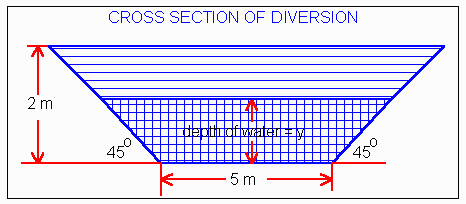An engineering study has determined that the relationship between the average speed and depth of water in the diversion in metres/sec is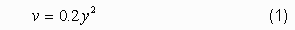This relationship has the following graph.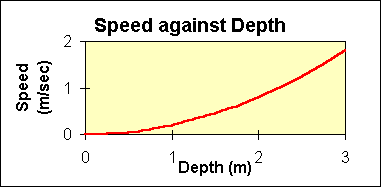Let us compute the maximum volume of water the diversion can handle. Suppose excess spring runoff lasts for a month. Water levels increase for 12 days and then decrease back to normal over the next 18 days. Suppose at the start (day 0) the water is just starting to enter the diversion (y = 0). From that time until day 12, the depth linearly increases to 2m (top of diversion), at which time the depth peaks. Then the depth linearly decreases, and on day 30, it reaches zero again. In other words, the graph of depth against time is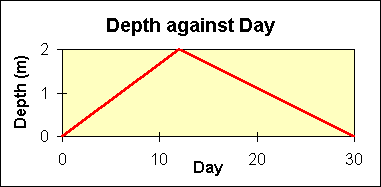## QUESTION:

What was the total volume of water that passed through the diversion during the 30 day period?

## SOLUTION:

### Step 1: Create an expression for total volume.

Using calculus, the instantaneous volume passing through a point in the diversion is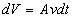as indicated in the following diagram.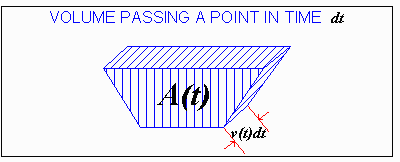The total volume passing through the diversion during the 30 day period is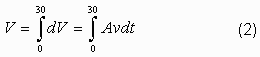Steps 2 to 6: Compute A and v as functions of t.

### Step 2: Express A (the cross-sectional area of water), as a function of y.

When the depth of water is y, the cross-sectional area of water is (recall the formula for the area of a trapezoid, and the geometry of a triangle)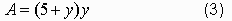### Step 3: Express y as a function of t.

y increases linearly for 12 days starting at 0m and ending at 2m. With the formula for a straight line,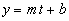we can use the two points (t = 0, y = 0) and (t = 12, y = 2) to determine the relationship,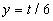where t is in days and y is in metres. Starting at day 12, the depth decreases for the next 18 days to zero. As above, we obtain the formula for a straight line between the points (t = 12, y = 2) and (t = 30, y = 0). This line is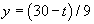Hence we have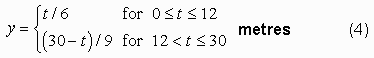### Step 4: Express A as a function of t.

A is related to y by equation (3), and y is related to t by equation (4).

Substituting equation (4) into (3), we obtain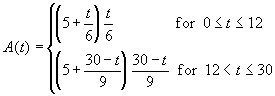Expanding the brackets: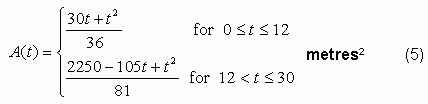### Step 5: Express v as a function of t.

v is related to y by equation (1), and y is related to tby equation (4). If we substitute equation (4) into (1), we obtain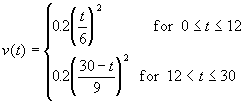Expanding the brackets: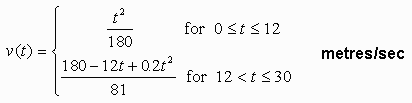### Step 6: Conversion of units.

Notice that the unit of time in the speed, v, is expressed in seconds but time everywhere else is expressed in days. To make the units coincide, let us express the speed in metres/day.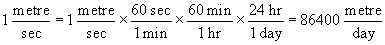So, the speed expressed in metres/day is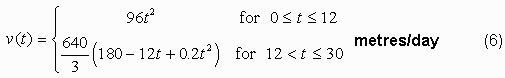### Step 7: Solving the integral.

From equation (2), break the integral into two time periods since there is a different relationship between A, v and t in each of these periods.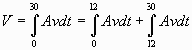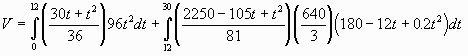Expanding the brackets and bringing constant factors outside the integral,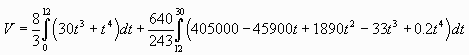Recall that for any n not equal to -1,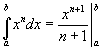Also, we can integrate an expression one term at a time. Integrating the expression for V: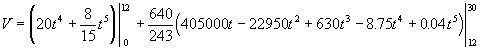We can now substitute the limits for the unknown, t, to obtain the answer.